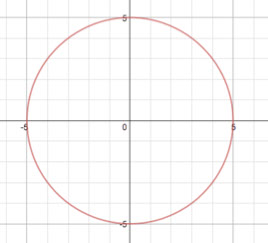# Parabolas Lesson 4 Episode 1 (Teachers)

### Making Sense

Keoni and Sasha make sense of the new situation in which the x-value is given and the y-value needs to be determined (instead of the other way around).

### Episode SupportsStudents’ Conceptual Challenges

Students may find it difficult to represent the distance from a point on the parabola to the directrix because the y-value is unknown.

After struggling with how to represent the unknown value of y and distances involving y [see 1:29 – 2:05], Sasha and Keoni abandon their approach and instead use the equation that they developed in Lesson 2, which is
b = √(4y).  [see 2:06].

Focus Questions

For use in a classroom, pause the video and ask these questions:

1. [Pause video at 0:58]. Sasha drew a vertical line from the x-axis up to the point on the parabola and then extended the line. How will that line segment be useful for their work?

2. [Pause video at 1:54]. Keoni said that the length one side of the right triangle is y – 1. Where is the distance represented by y? by By 1? By y – 1?

Supporting Dialogue

Engage your students in a discussion of the video:

1. Ask different students to share how the problem posed in this episode is alike and different from the problems from Lesson 3.

2. When one student responds to Focus Question 2, ask 2-3 two or three other students to restate the first student’s idea.

Math Extensions

Consider the circle below.

1. Find the y-value of points on the circle for x-values of 3, 4, and 2.

2. Find an equation for the y-value of a point on the circle for a general x-value.